Next: White Noise Up: APC591 Tutorial 7: A Previous: Introduction

# A Standard Wiener Process

A standard Wiener process (often called Brownian motion) on the interval
is a random variablethat depends continuously onand satisfies the following:

•
.
• For
,where
is a normal distribution with zero mean and unit variance. Because the normal distribution is used, the process is oftened referred to as Gaussian.
• For
,
and
are independent.

For use on a computer, we discretize the Wiener process with a timestep
as
In Matlab, an element of the distributionis obtained with the command randn''.

The article by Higham gives two equivalent Matlab programs to calculate a realization of a Wiener process. First bpath1.m:

%BPATH1  Brownian path simulation

randn('state',100)           % set the state of randn
T = 1; N = 500; dt = T/N;
dW = zeros(1,N);             % preallocate arrays ...
W = zeros(1,N);              % for efficiency

dW(1) = sqrt(dt)*randn;      % first approximation outside the loop ...
W(1) = dW(1);                % since W(0) = 0 is not allowed
for j = 2:N
dW(j) = sqrt(dt)*randn;   % general increment
W(j) = W(j-1) + dW(j);
end

plot([0:dt:T],[0,W],'r-')    % plot W against t
xlabel('t','FontSize',16)
ylabel('W(t)','FontSize',16,'Rotation',0)


Next bpath2.m:

%BPATH2  Brownian path simulation: vectorized

randn('state',100)          % set the state of randn
T = 1; N = 500; dt = T/N;

dW = sqrt(dt)*randn(1,N);   % increments
W = cumsum(dW);             % cumulative sum

plot([0:dt:T],[0,W],'r-')   % plot W against t
xlabel('t','FontSize',16)
ylabel('W(t)','FontSize',16,'Rotation',0)


These programs produce Figure 1.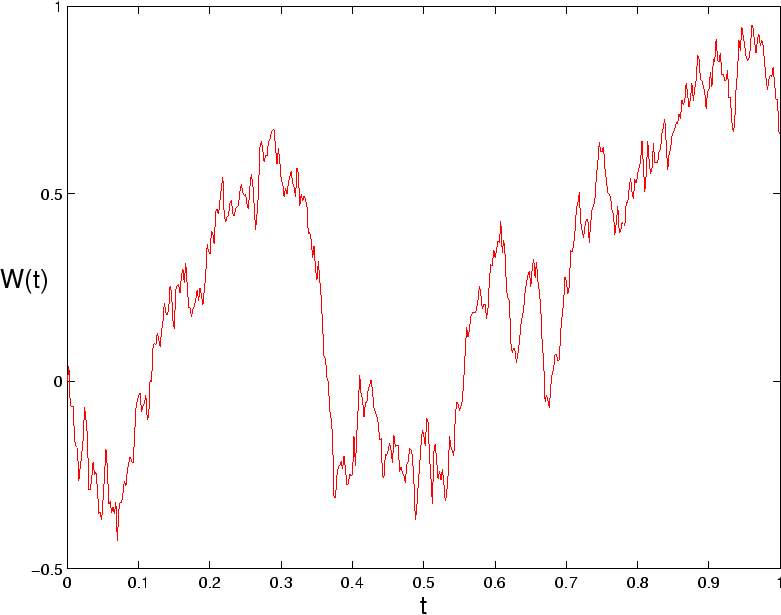Next: White Noise Up: APC591 Tutorial 7: A Previous: Introduction
Jeffrey M. Moehlis 2001-12-06